# SSC Reasoning Ability Practice Questions (Day-47)

Dear Aspirants, The SSC Exams are going on at national level. Millions of candidates have eagerly prepared for the ongoing SSC exams such as CGL, CHSL, MTS, CPO, JE etc. SSC exams are usually conducted by the Staff Selection Commission to recruit the eligible candidates for various Central government departments. Mostly the selection process for all the SSC exams consists of the single or double phase of written examination based on the examination. Due to that, the written exam consisted of a certain syllabus and exam pattern. By following those regulations, the written exam has been conducted through an online mode. Based on the latest updated exam pattern and syllabus, here we have drafted the Reasoning Ability questions on a daily basis. So aspirants start your practice session with us and mould your skill in a perfect shape.

Start Quiz

Direction (1): In the following question, select the number which can be placed at the sign of question mark (?) from the given alternatives.

1)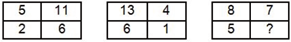(a) 3

(b) 4

(c) 6

(d) 7

Direction (2-3): How many triangles are there in the given figure?

2)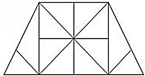(a) 18

(b) 20

(c) 24

(d) 28

3)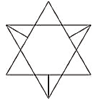(a) 12

(b) 14

(c) 16

(d) 20

Direction (4-5): In each of the following question below are given some statements followed by some conclusions. Taking the given statements to be true even if theyseem to be at variance from commonly known facts, read all the conclusions and then decide which of the given conclusion logically follows the given statements.

4) Statements:

I) Some pens are pencils.

II) All pencils are erasers.

III) Some erasers are cups.

Conclusions:

I) Some pens are cups.

II) Some pencils are cups.

III) Some cups are pencils.

IV) Some erasers are pens.

Only conclusion (II) follows

Only conclusion (IV) follows

Only conclusion (I) follows

No conclusion follows

5) Statements:

I) Some pens are cups.

II) No cups are plates.

Conclusions:

I) Some pens are not plates.

II) All pens are plates.

III) Some plates are not pens.

(a) Only conclusion (I) and (II) follow

(b) Only conclusion (I) follows

(c) Only conclusion (II) and (III) follow

(d) No conclusion follows

6) Form the given, which answer figure can be formed by folding the figure given in the question?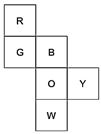(a)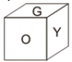(b)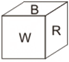(c)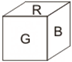(d)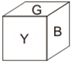7) From the given options, which answer figure can be formed by folding the figure given in the question?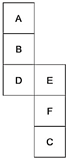(a)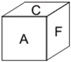(b)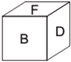(c)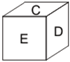(d)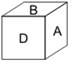8) In the given figure, which number represents houses which are red and tall?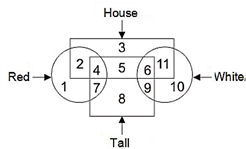(a) 7

(b) 4

(c) 8

(d) 11

9) In the given figure, how many males shoes are not of clothes?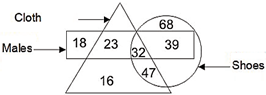(a) 71

(b) 39

(c) 79

(d) 23

10) In the given figure, which number represents white glass which is not cup?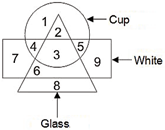(a) 2

(b) 3

(c) 6

(d) 4

5 + 2 + 6 + 11 = 24

13 + 6 + 1  + 4 = 24

8 + 5 + 4 + 7 = 24

24 triangles

14 triangles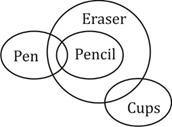I) × II) × III) ×      IV) √

Only conclusion IV follows.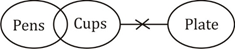I) √ II) × III) ×

Only conclusion I follows.

Opposite faces are –

R → O

B → W

G → Y

∴ Option (c) can be formed.

Opposite faces are

B → F

E → C

A → D

Option (a) Can be formed.

4 represents houses which are red and tall.

39 male shoes are not of clothes.